Question

4. For the following laboratory data, calculate the weight of moist soil, moist unit weight, moisture...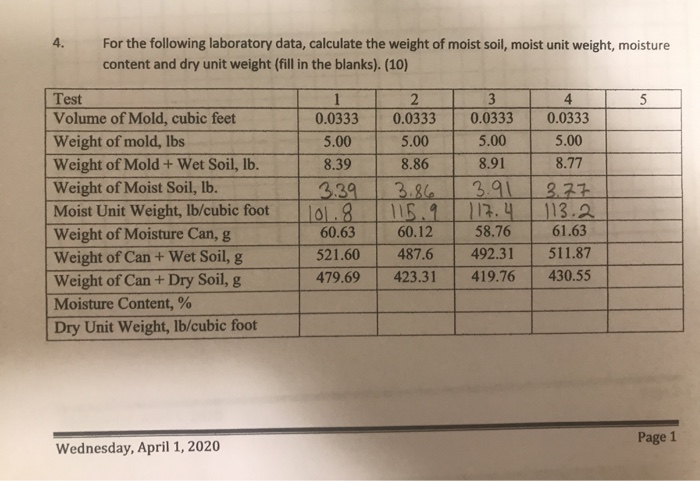4. For the following laboratory data, calculate the weight of moist soil, moist unit weight, moisture content and dry unit weight (fill in the blanks). (10) Test Volume of Mold, cubic feet Weight of mold, lbs Weight of Mold + Wet Soil, lb. Weight of Moist Soil, lb. Moist Unit Weight, lb/cubic foot Weight of Moisture Can, g Weight of Can + Wet Soil, g Weight of Can+Dry Soil, g Moisture Content, % Dry Unit Weight, lb/cubic foot | 0.0333 0.0333 0.0333 0.0333 5.00 5.00 5.00 5.00 8.39 8.868 .91 8.77 3.39 3.86 3.91 13. 77 1101.8 116.91 117.4 1113.2 60.6360.12 58.76 61.63 521.60 487.6 492.31 1 511.87 479.69 423.31 419.76 430.55 Page 1 Wednesday, April 1, 2020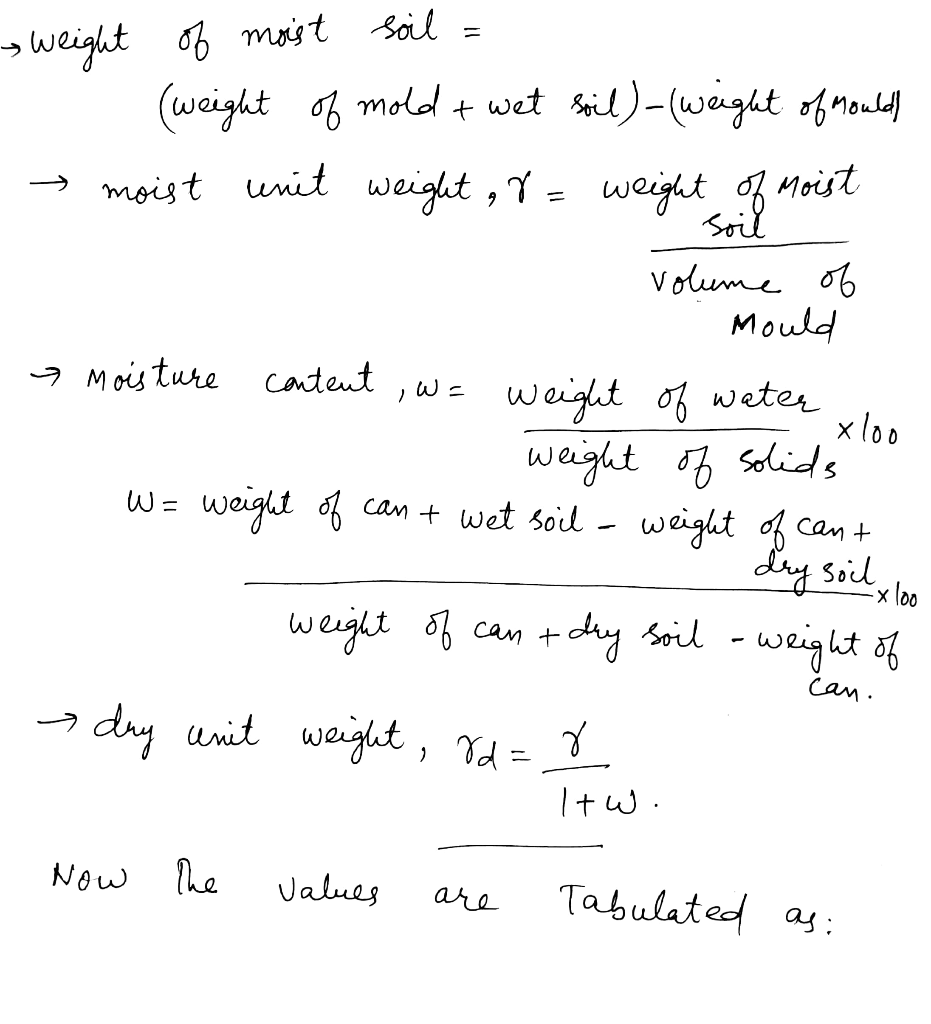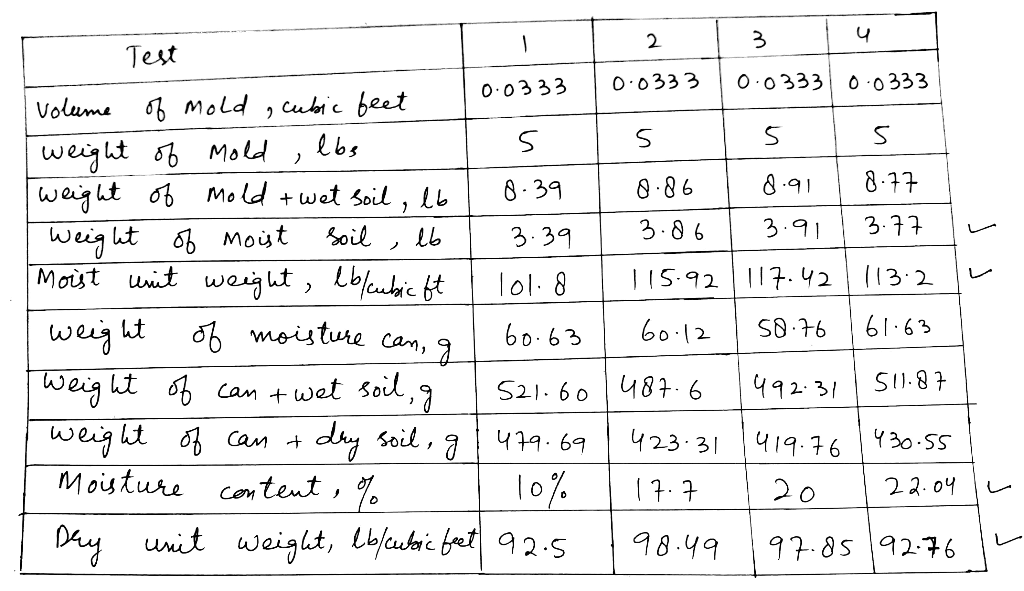Earn Coins

Coins can be redeemed for fabulous gifts.

Similar Homework Help Questions
• The following information is given for a moist soil. What would be approximately the dry unit...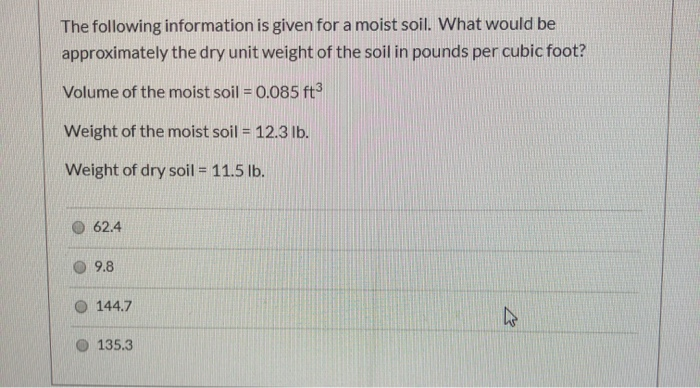The following information is given for a moist soil. What would be approximately the dry unit weight of the soil in pounds per cubic foot? Volume of the moist soil = 0.085 ft3 Weight of the moist soil = 12.3 lb. Weight of dry soil = 11.5 lb. 0 62.4 9.8 144.7 135.3

• Problem 1(25%): The moist weight of 0.2 f of a soil is 23 Ib. The moisture...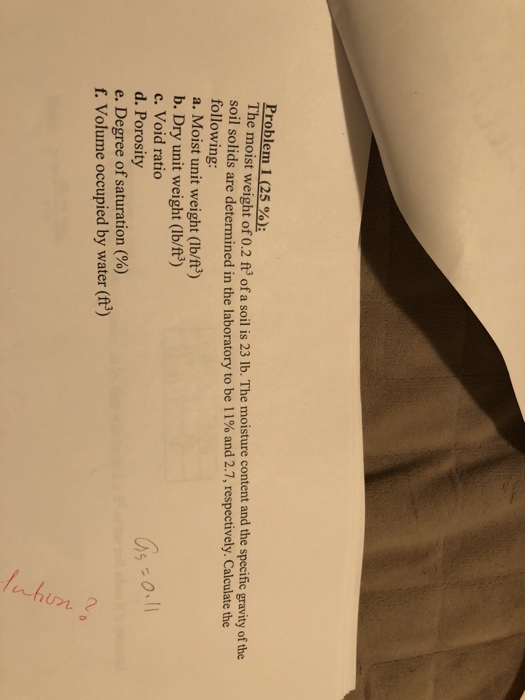Problem 1(25%): The moist weight of 0.2 f of a soil is 23 Ib. The moisture content and the specific gravity of the soil solids are determined in the labortory to be 1 1 % and 2.7, respectively. Calculate the following: a. Moist unit weight (Ib/ft3) b. Dry unit weight (lb/f3) c. Void ratio d. Porosity e. Degree ofsaturation (%) 5 f. Volume occupied by water (ft3)

• A field unit weight determination test for the soil described in Problem 6.6 yielded the following...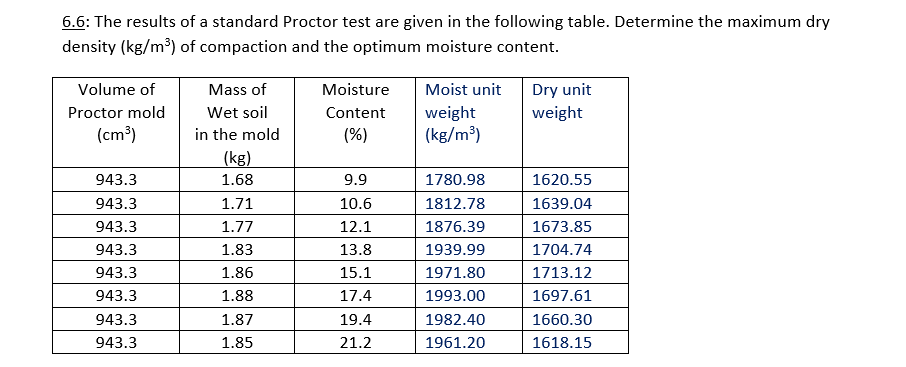A field unit weight determination test for the soil described in Problem 6.6 yielded the following data: moisture content=10.5% and moist density=1705 kg/m3. Determine the relative compaction. 6.6: The results of a standard Proctor test are given in the following table. Determine the maximum dry density (kg/m²) of compaction and the optimum moisture content. Volume of Proctor mold (cm) Moisture Content (%) Moist unit weight (kg/m3) Dry unit weight 943.3 943.3 943.3 943.3 943.3 943.3 943.3 943.3 Mass of Wet...

• For a soil sample, the wet unit weight is 120 lb/ft3, and the moisture content is...

For a soil sample, the wet unit weight is 120 lb/ft3, and the moisture content is 20%. What is the dry unit weight of this soil sample? answer choices 120 lb/ft3 110 lb/ft3 105 lb/ft3 100 lb/ft3

• The following results were obtained from standard Proctor compaction tests. Test ID Mass of moist soil...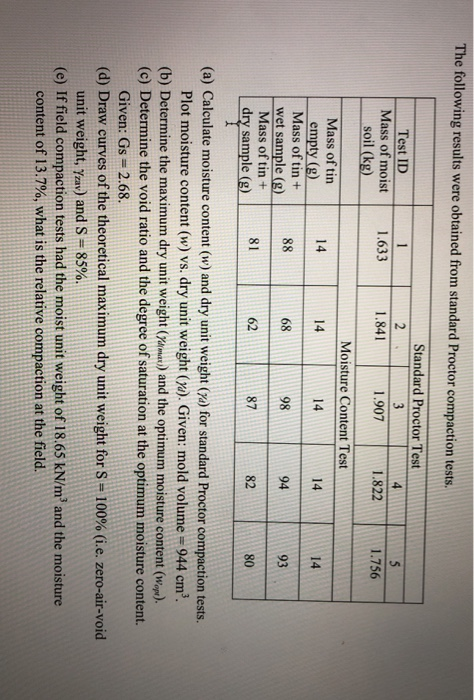The following results were obtained from standard Proctor compaction tests. Test ID Mass of moist soil (kg) 1 1.633 Standard Proctor Test 2 . 1.841 1.907 1.822 1.756 Moisture Content Test 14 14 Mass of tin empty (g) Mass of tin + wet sample (g) Mass of tin + dry sample (g) 98 62 878280 (a) Calculate moisture content (w) and dry unit weight (ya) for standard Proctor compaction tests. Plot moisture content (w) vs. dry unit weight (yd). Given:...

• B. The moist weight of 0.028 m3 of a soil is 52 kg. The moisture content...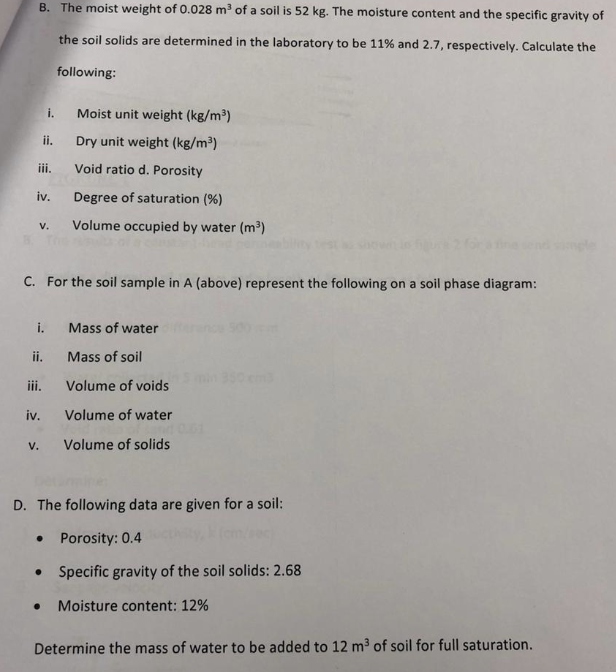B. The moist weight of 0.028 m3 of a soil is 52 kg. The moisture content and the specific gravity of the soil solids are determined in the laboratory to be 11% and 2.7 , respectively. Calculate the following: Moist unit weight (kg/m3) i. Dry unit weight (kg/m3) ii. Void ratio d. Porosity iii. iv. Degree of saturation (%) Volume occupied by water (m3) v. ngle C. For the soil sample in A (above) represent the following on a soil...

• Problem 1 (3 points) The results of a standard Proctor test are given in the following...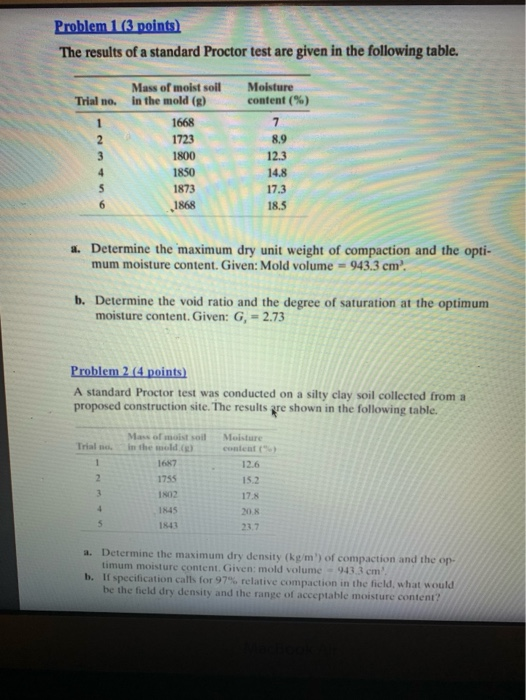Problem 1 (3 points) The results of a standard Proctor test are given in the following table. Moisture Mass of moist soil in the mold (R) Trial no. 1668 1723 1800 1850 1873 8.9 12 1868 a. Determine the maximum dry unit weight of compaction and the opti- mum moisture content. Given: Mold volume = 943.3 cm'. b. Determine the void ratio and the degree of saturation at the optimum moisture content. Given: G, = 2.73 Problem 2 (4 points)...

• answer q4 based on 2 4. For the soil in question 2, how much glucose must be added on a dry weight soil basis if the gl...answer q4 based on 2 4. For the soil in question 2, how much glucose must be added on a dry weight soil basis if the glucose amendment is 10% 2. How much water must be added to 100 g of a moist soil at an initial moisture content of 10%, so that the final soil moisture content is 15%? e(Initial) = 0.10 m(Final) = 100 d ? Solution 100 d 0.1 1.1 d 100 d 90.9 e(Final) = 0.15...

• Question one Find water content, weight moist soil & can = 25 g Weight can =...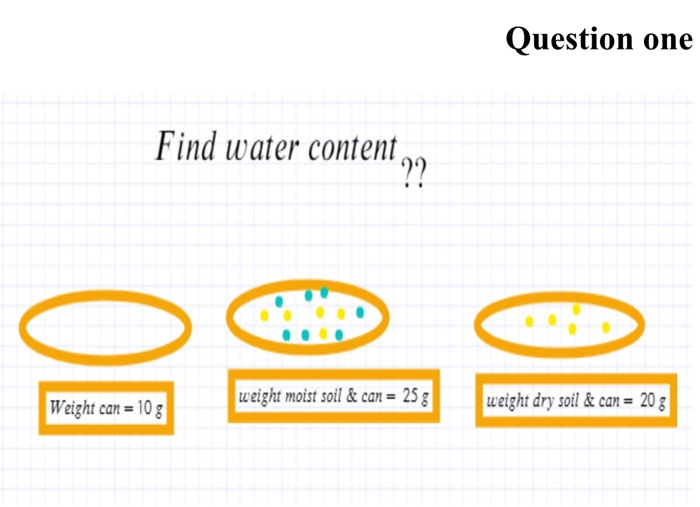Question one Find water content, weight moist soil & can = 25 g Weight can = 10g weight dry soil & can = 20 g 7. The grain size analysis for a soil is given as: Sieve No. Grain Size (mm) Passing 0.42 0.15 0.075 200 a) Plotagrain-sine distribution curve b) Determine D D and De from the grain size distribution curve c) Calculate the uniformity coefficient, d) Calculate the coefficient of curvature. Ce c) Classify the soil using the...

• QUESTION 2 A sand cone test has been performed in a recently compacted fill. The test...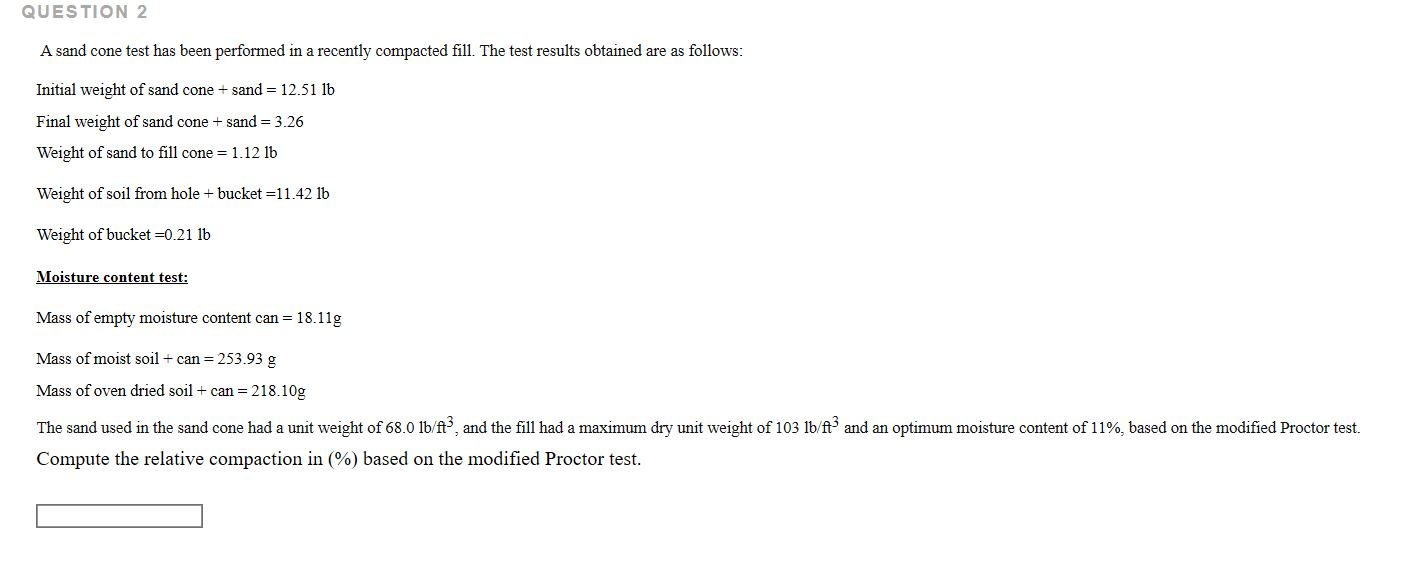QUESTION 2 A sand cone test has been performed in a recently compacted fill. The test results obtained are as follows: Initial weight of sand cone + sand = 12.51 lb Final weight of sand cone + sand = 3.26 Weight of sand to fill cone = 1.12 lb Weight of soil from hole + bucket =11.42 lb Weight of bucket =0.21 lb Moisture content test: Mass of empty moisture content can = 18.11g Mass of moist soil + can...